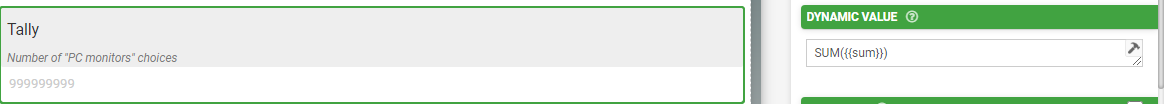# Counting Specific Choice in Table

I would like to count the number of times a specific choice gets picked in a repeating table. How would i do this?

• Try using the TOLIST() function and add an optional parameter that only adds the field to the list if {{fieldName}} = "specific choice". This will create a list with all of the instances of your specific chose. So for example, if there are 5 rows for your specified chose, the list will have the specified chose 5 times.

You can then use the SUM function to add all the instances is in your list.

You would need to update the formula accordingly but it would look something like this:

SUM(TOLIST({{fieldName}}, ', ', '{{this}} = specified chose'))

• This doesn't work because the TOLIST function expects a single value, not a repeat table like you're giving it from the repeating table. I'm trying to solve the same problem. Is there another way?

• You can do this with SELECTED... see below. The formula I use in my hidden field inside the table is:

IF((SELECTED({{choicesfield}}, 'PC monitors')),1,0)Then outside the table use another field to sum up all of these fields in the tableLooks like this in the app :)